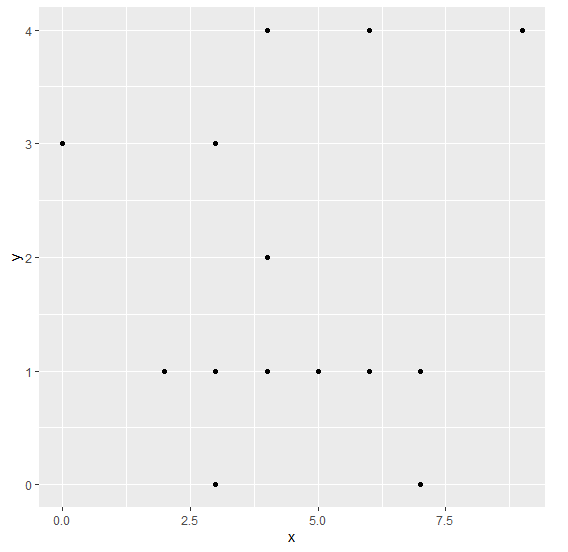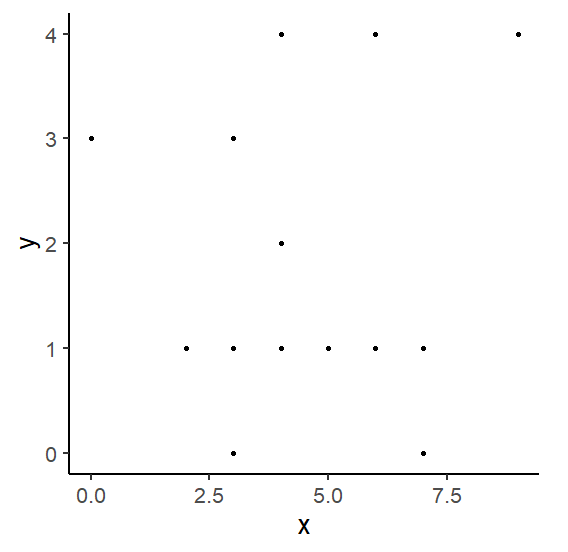# Create ggplot2 graph with darker axes labels, lines and titles in R

To create a ggplot2 graph with darker axes labels, darker lines, and dark titles, we can use theme_classic function of ggplot2 package with base_size argument set to a larger value.

For Example, if we have a data frame called df that contains two columns say x and y then we can create the scatterplot between x and y using ggplot2 with darker axes labels, darker lines, and dark titles by using the below command −

ggplot(df,aes(x,y))+geom_point()+theme_classic(base_size=22)

## Example

Following snippet creates a sample data frame −

x<-rpois(20,5)
y<-rpois(20,2)
df<-data.frame(x,y)
df

The following dataframe is created

   x y
1 9 4
2 2 1
3 4 4
4 7 0
5 7 0
6 3 1
7 3 1
8 3 1
9 3 0
10 6 1
11 5 1
12 6 4
13 6 1
14 4 1
15 7 1
16 3 3
17 0 3
18 4 4
19 4 2
20 3 1

To load ggplot2 package and create scatterplot between x and y on the above created data frame, add the following code to the above snippet −

x<-rpois(20,5)
y<-rpois(20,2)
df<-data.frame(x,y)
library(ggplot2)
ggplot(df,aes(x,y))+geom_point()

## Output

If you execute all the above given snippets as a single program, it generates the following Output −To create scatterplot between x and y with darker axes labels, darker lines, and dark titles on the above created data frame, add the following code to the above snippet −

x<-rpois(20,5)
y<-rpois(20,2)
df<-data.frame(x,y)
library(ggplot2)
ggplot(df,aes(x,y))+geom_point()+theme_classic(base_size=20)

## Output

If you execute all the above given snippets as a single program, it generates the following Output −